For a full list of BASHing data blog posts see the index page.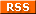# Character equivalence classes 1: search and replace

Searching for "something like". A POSIX equivalence class is a group of characters that can be treated as the same character in your computer's locale. The class is written [=x=], which means "any character that's something like x".

For example, if I grep for the character "a" in the string "caáäb", I find only "a". If I grep for "something like a" or "something like á" or "something like ä" I get everything except "c" and "b":

grep -o "a" <<<"caáäb" | paste -s -d " "
grep -o "[[=a=]]" <<<"caáäb" | paste -s -d " "
grep -o "[[=á=]]" <<<"caáäb" | paste -s -d " "
grep -o "[[=ä=]]" <<<"caáäb" | paste -s -d " "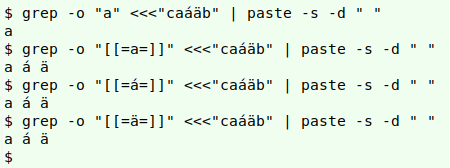I can also replace the character within the equivalence class expression with a command output. Here I'm repeating those last three grep commands with the outputs from printf-ing the characters' hexadecimal representations:

grep -o "[[=\$(printf "\x61")=]]" <<<"caáäb" | paste -s -d " "
grep -o "[[=\$(printf "\xc3\xa1")=]]" <<<"caáäb" | paste -s -d " "
grep -o "[[=\$(printf "\xc3\xa4")=]]" <<<"caáäb" | paste -s -d " "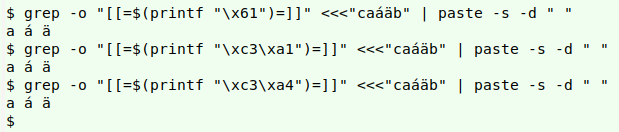Below I'm searching for "something like" "c" or "e", then "C" or "É". These searches have exactly the same result. An equivalence class includes both the lowercase and uppercase versions of a character in the class.

grep -o "[[=c=][=e=]]" <<<"Émile's cat is in the Café Forèt" \
| paste -s -d " "

grep -o "[[=C=][=É=]]" <<<"Émile's cat is in the Café Forèt" \
| paste -s -d " "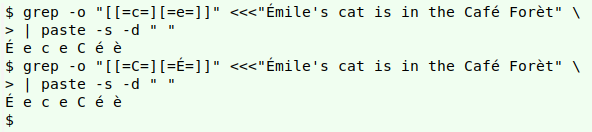One "gotcha" in using equivalence classes for searching is that they can't be used at the start or end of a range in a bracket expression:

grep -o "[a-e]" <<<"Émile's cat is in the Café Forèt" \
| paste -s -d " "

grep -o "[a-[=e=]]" <<<"Émile's cat is in the Café Forèt" \
| paste -s -d " "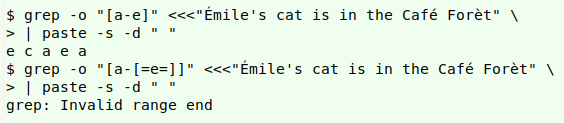As it says in the POSIX specifications:

An equivalence class expression used as a starting or ending point of a range expression produces unspecified results. An equivalence class can be used portably within a bracket expression, but only outside the range.

Replacing "something like". Equivalence classes can be used for replacing, but not easily. To begin with, the GNU tr command doesn't handle multi-byte characters very well yet (I'm using coreutils version 8.3) and gives inconsistent results:

tr -d "é" <<<"café"
tr "[:lower:]" "[:upper:]" <<<"café"
tr "é" "e" <<<"café"
tr "[=e=]" "e" <<<"café"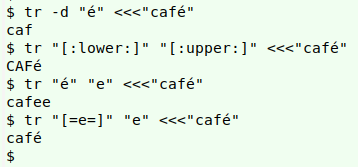Equivalence classes also can't be used for regex matching or replacement with GNU AWK. Fortunately, GNU sed understands the "something-like" class:

sed 's/[[=e=]]/e/g' <<<"Café Forèt"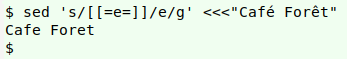In fact, with GNU sed you can even use hexadecimal, octal and Unicode representations of a character within the [=x=] expression. Here I'm doing it with [=e=], and note that the second and third commands are unquoted:

sed "s/[[=\x65=]]/e/g" <<<"Café Forèt"
sed s/[[=\$'\145'=]]/e/g <<<"Café Forèt"
sed s/[[=\$'\u0045'=]]/e/g <<<"Café Forèt"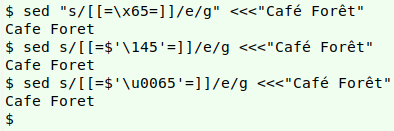Suppose you have a word with several different multi-byte characters that you want to ASCIIfy, i.e. have plain characters in place of the ones with diacritical marks. With sed you could do the replacements one by one:

sed 's/[[=s=]]/S/;s/[[=e=]]/e/;s/[[=c=]]/c/' <<<"Štěpnička"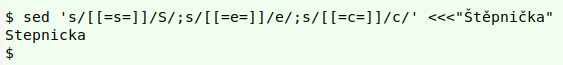Notice that I'm replacing the capital letter "Š" with a capital "S". If there were also a lowercase "š" or "s" in the word, it too would get replaced with "S", because the character equivalence class [=s=] ignores case.

But sed also has a "y" option that can be used to replace a sequence of characters. In other words, replace the first character in the search sequence with the first character in the replacement sequence, and so on:

sed 'y/Šěč/Sec/' <<<"Štěpnička"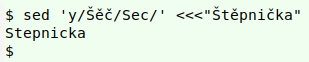Alas, this doesn't work with "something-like" expressions:

sed 'y/[[=s=][=e=][=c=]]/Sec/' <<<"Štěpnička"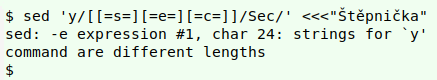...and I don't know of any workarounds.

Part 2 of this series explores what "something like" actually means.

Last update: 2020-06-17
The blog posts on this website are licensed under a